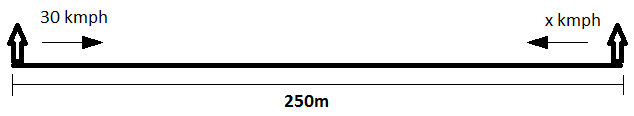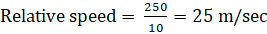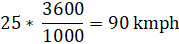Question of The Day18-07-2022

Two boys are standing 250 m away from each other on their bikes. They start to travel towards each other and cross after 10 sec.  If the first boy is travelling at a speed of 30 kmph then find the speed of the second boy.

Correct Answer : d ) 55 kmph

Explanation :

Let the speed of the second boy be x kmph.

Total distance needed to be travelled to cross each other = 250 m

Time taken by boys to cross each other = 10 secSince, boys are travelling towards each other.

Thus, the relative speed of boys = (30 + x) kmphConverting relative speed in kmphRelative speed = 90 kmph

(30 + x) kmph = 90 kmph

So, x = 60 kmph

Thus, the speed of the second boy = 60 kmph

Hence, (e) is the correct answer.0